#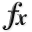N-D Test Functions Q¶

class go_benchmark.Qing(dimensions=2)

Qing test objective function.

This class defines the Qing global optimization problem. This is a multimodal minimization problem defined as follows: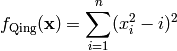Here,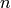represents the number of dimensions and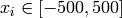for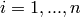.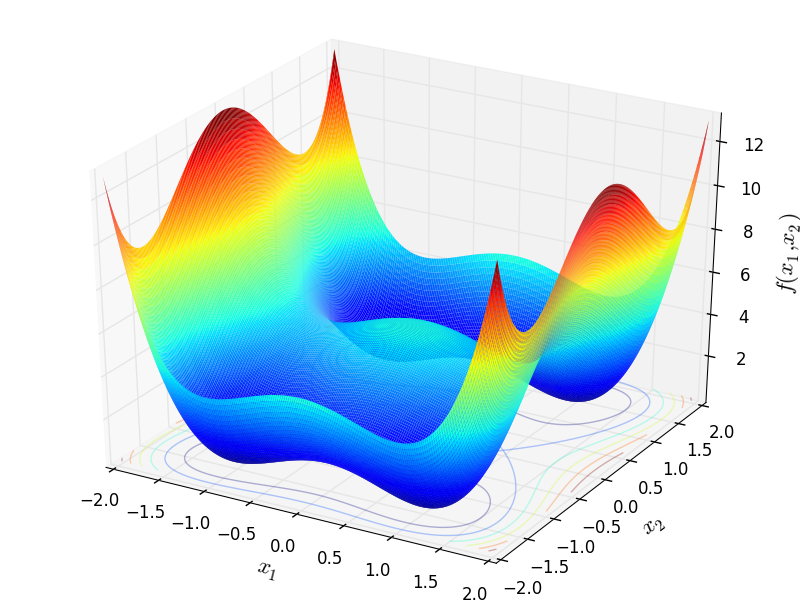Two-dimensional Qing function

Global optimum: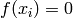for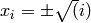forThis class defines the Quadratic global optimization problem. This is a multimodal minimization problem defined as follows: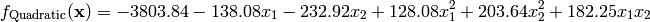Here,represents the number of dimensions andfor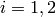.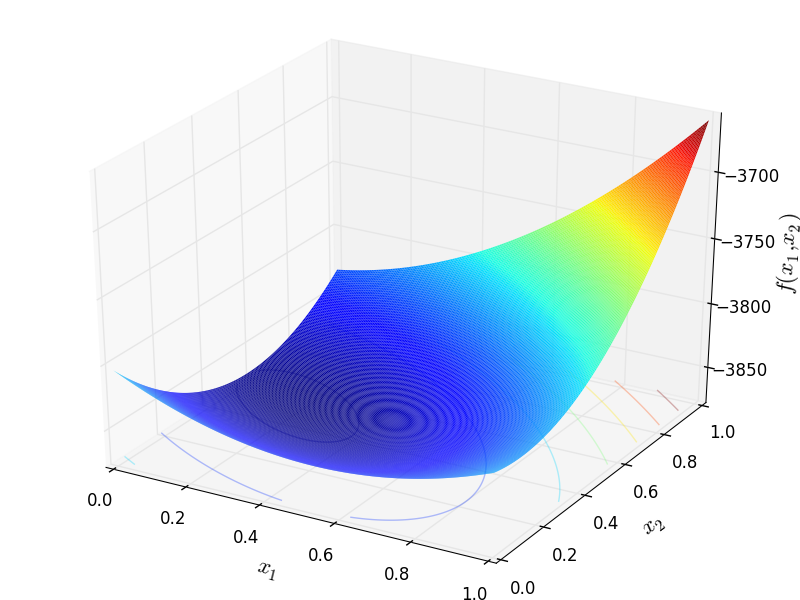Global optimum:forclass go_benchmark.Quintic(dimensions=2)

Quintic test objective function.

This class defines the Quintic global optimization problem. This is a multimodal minimization problem defined as follows:Here,represents the number of dimensions andfor.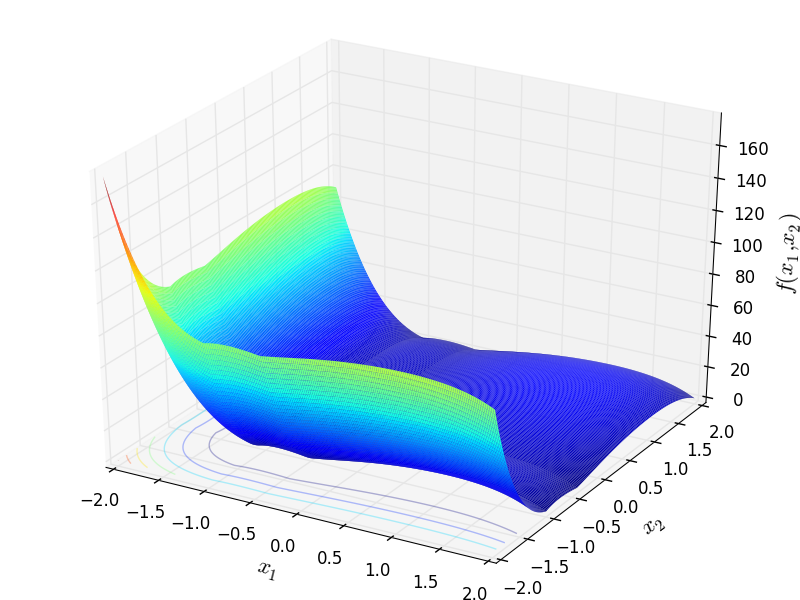Two-dimensional Quintic function

Global optimum:for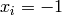for#### Previous topic

N-D Test Functions P

#### Next topic

N-D Test Functions R